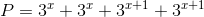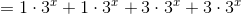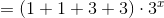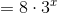# GMAT Math : Calculating the perimeter of a rectangle

## Example Questions

### Example Question #1 : Quadrilaterals

A rectangle has a length ofand width of. Solve for the perimeter.Explanation: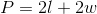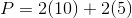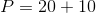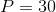### Example Question #8 : Quadrilaterals

A farmer decides to build a fence two feet around his rectangular field. The field isfeet long andfeet wide. How long should the fencing be in order to build the fence around the field?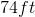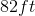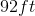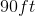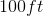Explanation:

The fence is 2 feet around the field. Therefore the area actually enclosed by the fence is 4 feet longer and 4 feet wider than the field.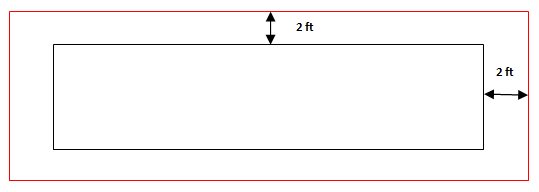The length of fencing needed is the perimeter of the area enclosed which is calculated as follows: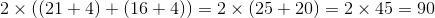The fencing should be 90 feet.

### Example Question #9 : Quadrilaterals

If a rectangle has an area ofand a length of, what is its perimeter?Explanation:

To find the perimeter, we need the length and the width. We are only given the length, so first we must find the width using the given area: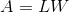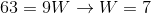The perimeter is simply two times the length plus two times the width, so we can now use the known length and width to calculate the perimeter: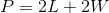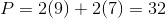### Example Question #10 : Quadrilaterals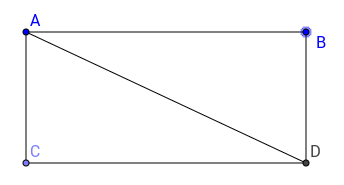The diagonal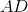of the rectangle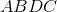iscm long and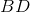iscm long. What is the perimeter of the rectangle?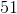Explanation:

We can see that the hypotenuse AD of triangle ABD is 15 cm. We should check whether ABD is a Pythagorean Triple, whose sides are in the ratio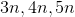, whereis a constant. Since AD is 15 and BD is 9, the triangle must be a Pythagorean Triple with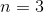, therefore AB must be 12 cm long. Now we know all the lengths of the sides of our triangle, we can find the perimeter which will be given by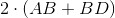, which gives us 42, our final answer.

### Example Question #2 : Calculating The Perimeter Of A RectangleThe area of rectangleis, and the diagonal has length. What is the perimeter of rectangle?Explanation:

Given the information we are provided, we could set up an quadratic equation, however, this equation would be really complicated to solve, instead we should try to find whether the diagonal of this rectangle can be the hypotenuse of Pythagorean triangle. The hypotenuse AD of triangle ADC, has length 10, therefore if it is a Pythagorean Triple, its other lengths must be 6 and 8, since the sides are in ratiowhereis a constant. By testing, we see that if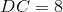and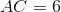we get an area of 48 for this rectangle, by multiplying DC by AC. This is the original area of our rectangle, therefore, these must be the right lenghts and the final answer is given by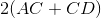, which is 28.

Alternatively, we could have found the missing lengths with trial and error, by doing so, we would have picked length for which, the area is 48 and tried to see whether the diagonal would remain 10, until we get the working set 6 and 8.

### Example Question #3 : Calculating The Perimeter Of A Rectangle

The width of a rectangle is twice the length. Find an equation representing the perimeter.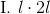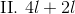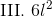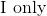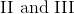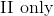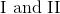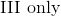Explanation:

The perimeter of a rectangle can be found by adding up all the sides: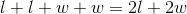We are told the width of the rectangle is twice its length so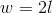, inserting this into our equation for the perimeter leaves us with: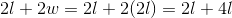, which is II

### Example Question #1 : Calculating The Perimeter Of A Rectangle

Find the perimeter of a rectangle whose width isand length is.Explanation:

To solve, simply use the formula for the perimeter of a rectangle: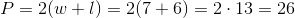### Example Question #5 : Calculating The Perimeter Of A Rectangle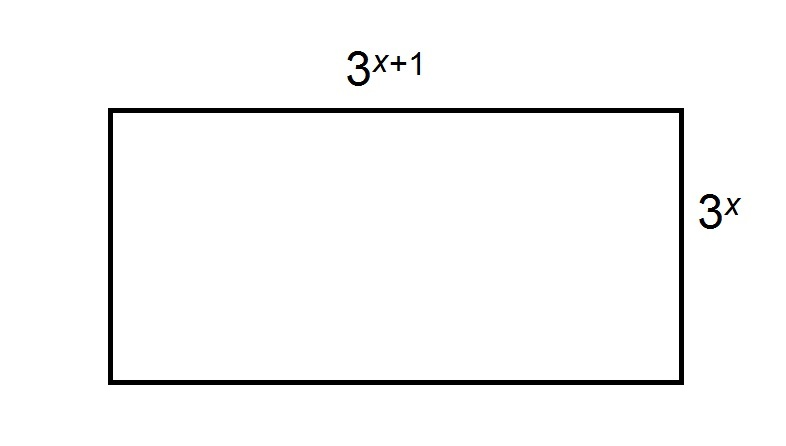Note: figure NOT drawn to scale

Give the perimeter of the above rectangle.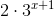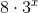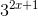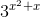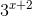Explanation:

The perimeter of a rectangle is the sum of the lengths of its sides. Since a rectangle has two pairs of sides of the same lengths, this will be